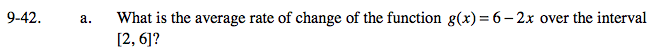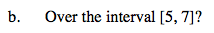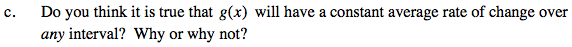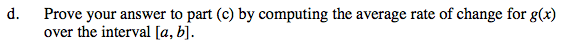### Home > PC > Chapter 9 > Lesson 9.1.3 > Problem9-42

9-42.$\frac{g(6) - g(2)}{6-2}$$\frac{g(7) - g(5)}{7-5}$Yes, because it is linear.$\frac{g(b) - g(a)}{b-a} = \frac{6 - 2b - (6 - 2a)}{b - a} =$